# Coastline Fractal Dimensions

Fractal dimensions of coastlines of all countries, dependencies, and territories

## Details

A country’s coastline has no well-defined length, as its length depends on the length of the ruler used to estimate it. A shorter ruler allows for more accuracy in measuring complex, irregular coastlines; thus, as the ruler length decreases, the coastline length becomes theoretically infinite. This is known as the “Coastline Paradox”, which can be attributed to the fractal-like property of coastlines. A coastline’s fractal dimension provides a quantitative index of complexity, measuring how fast the estimated coastline length increases with respect to a smaller and smaller scale at which it is measured. This dataset was created to calculate and compare the fractal dimensions of coastlines around the world. This dataset contains the fractal dimensions of coastlines of 249 countries, dependencies, and territories, generated using ResourceFunction[“CoastlineFractalDimension”]. If a country is landlocked, its fractal dimension is 0.

## Examples

### Basic Examples (3)

 In:=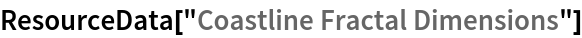In:=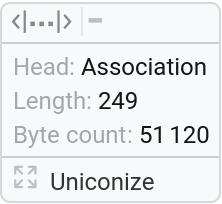Return the fractal dimension of the United Kingdom:

 In:=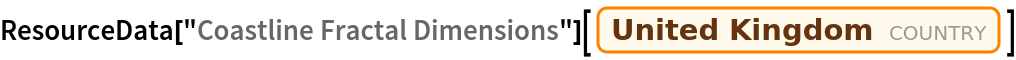Out=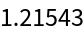Get a Dataset ranking all countries, dependencies, and territories by their coastline fractal dimension:

 In:=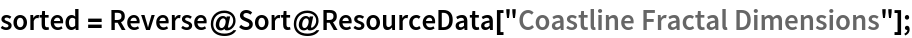In:=Out=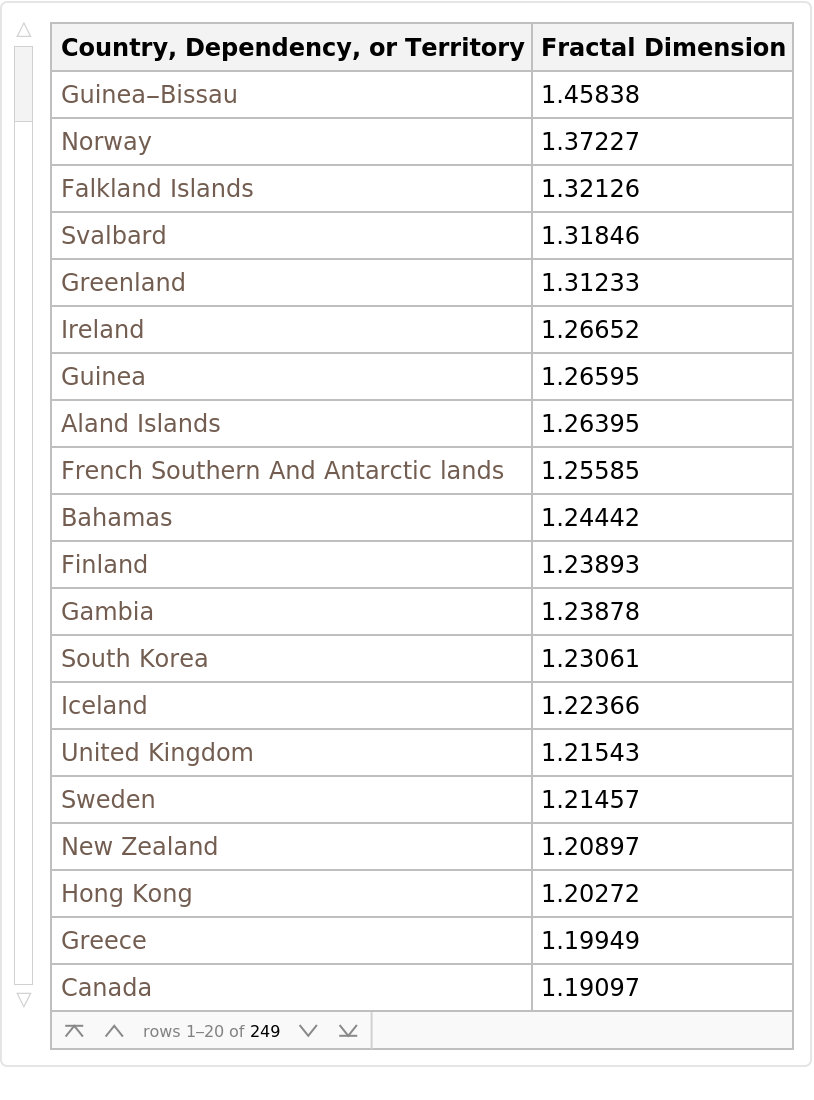### Visualizations (2)

Generate a world map with each country, dependency, and territory labeled with a pop-up window with its fractal dimension:

 In:=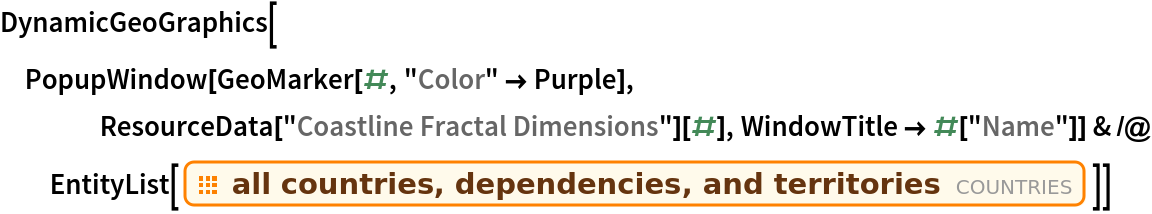Out=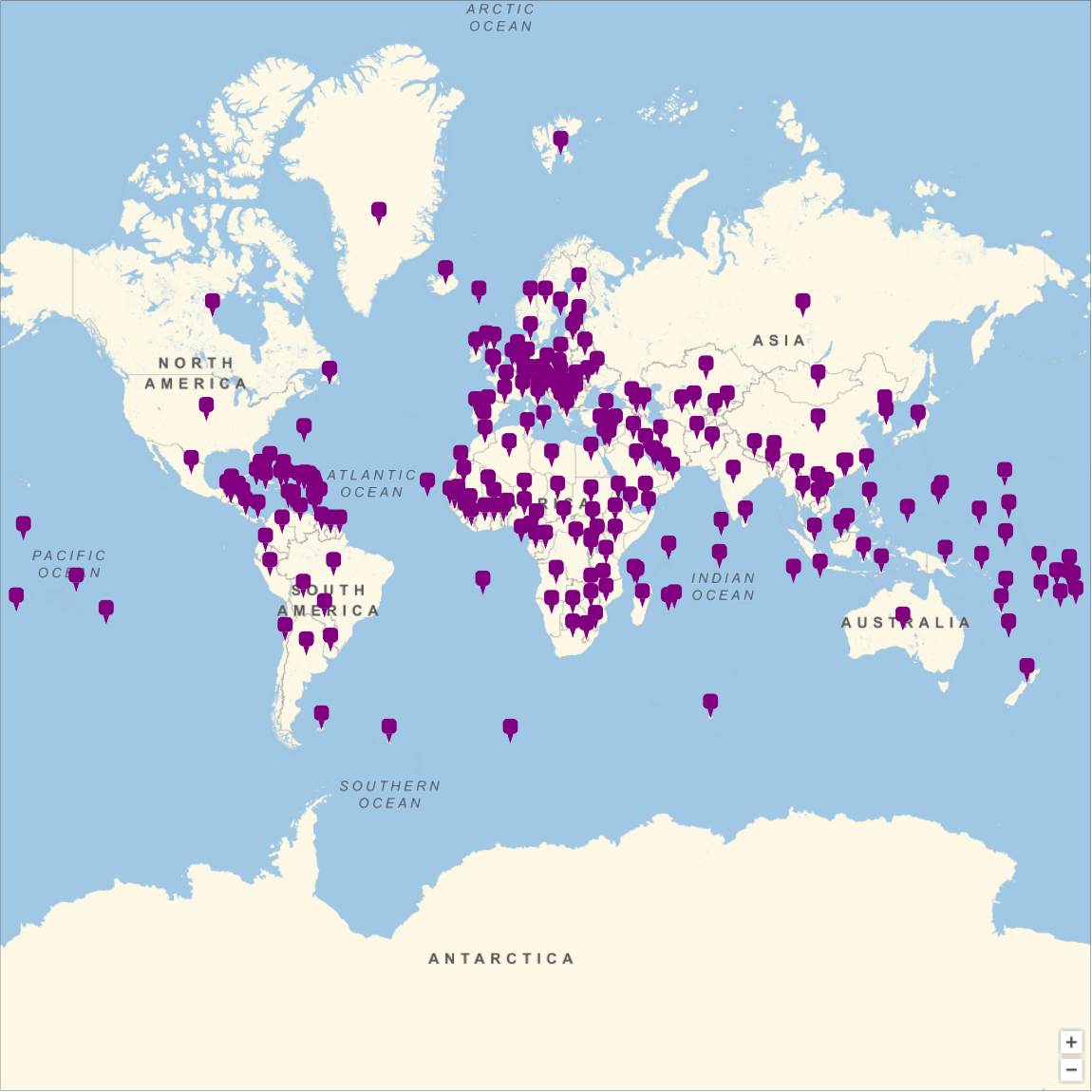Compare coastline fractal dimensions around the world:

 In:=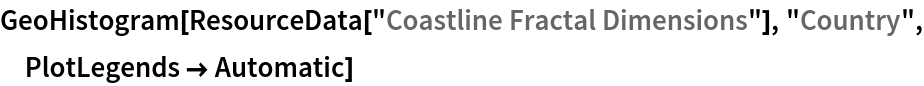Out=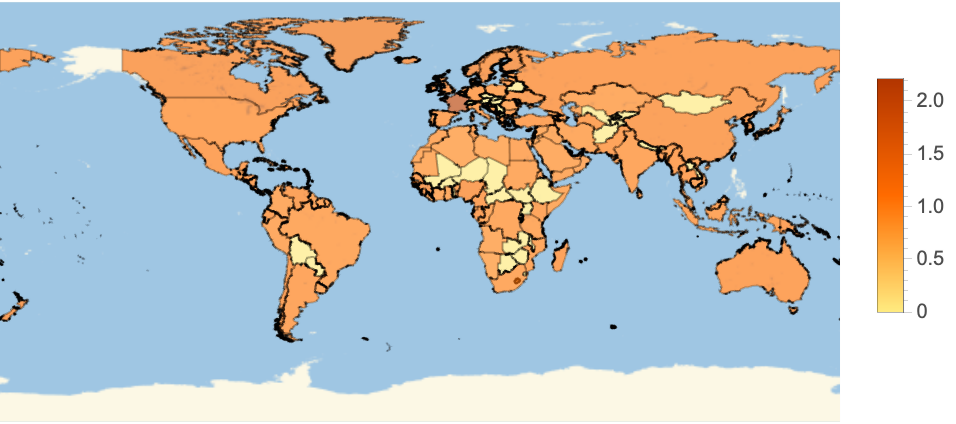Generate a table to visualize the five countries, dependencies, and/or territories with the highest fractal dimensions:

 In:=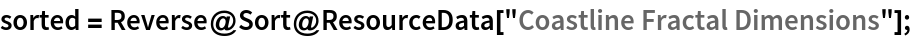In:=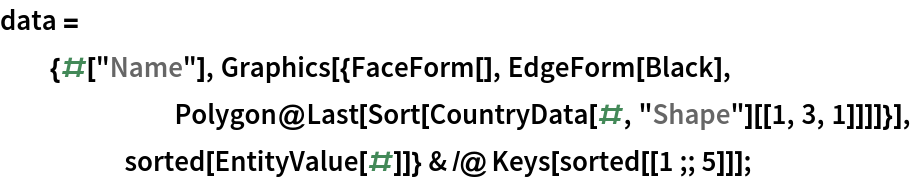In:=Out=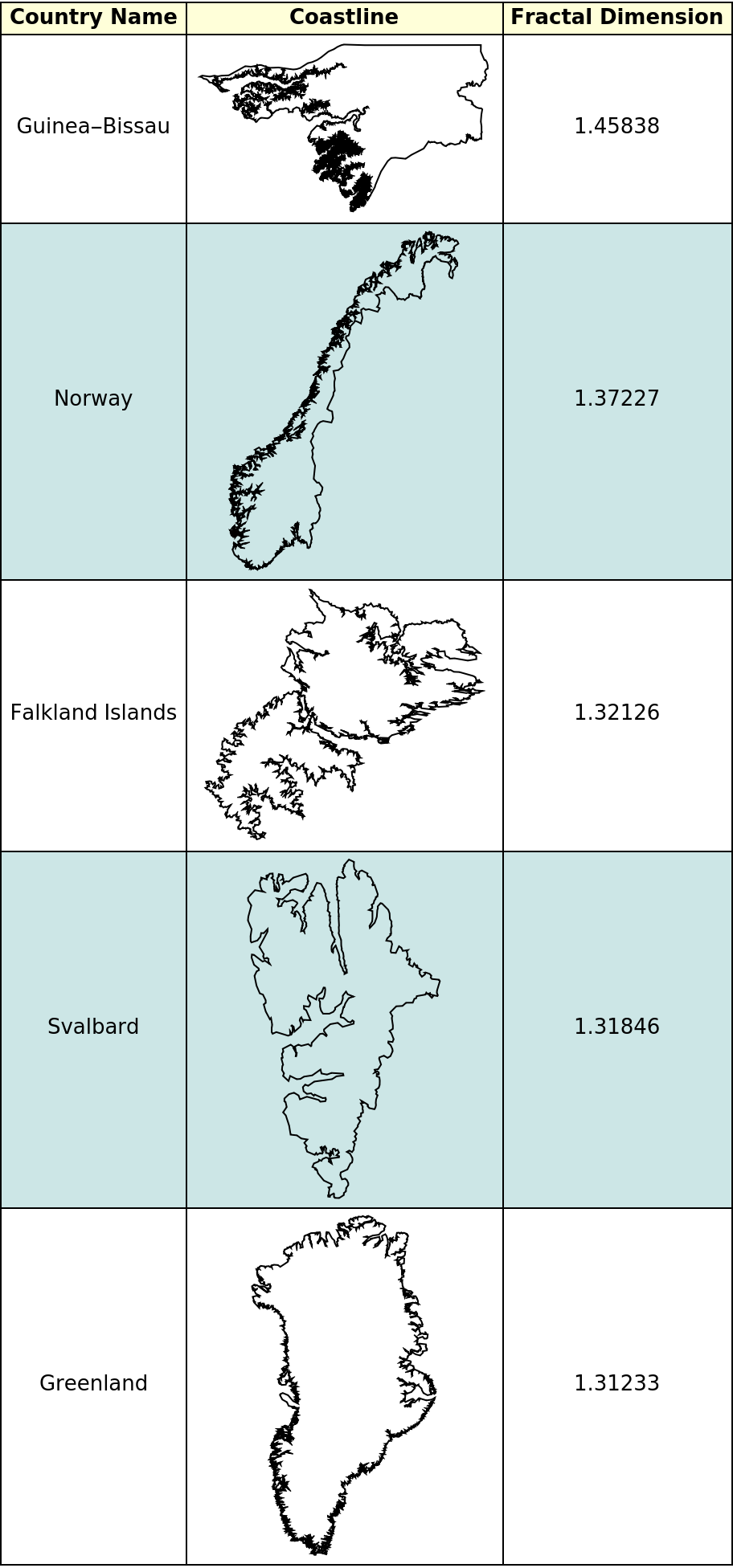Wolfram Summer Camp, "Coastline Fractal Dimensions" from the Wolfram Data Repository (2021)